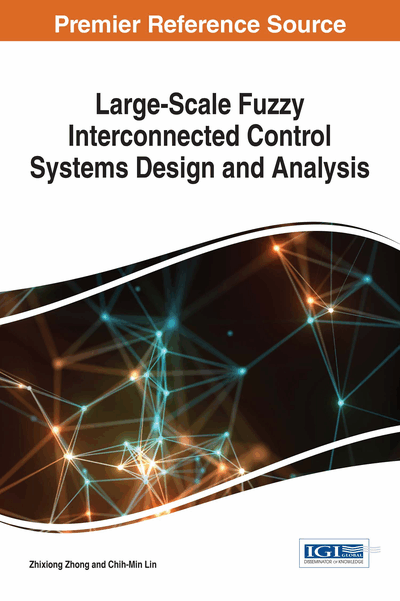# Sliding-Mode Control of Large-Scale Fuzzy Interconnected Systems

DOI: 10.4018/978-1-5225-2385-7.ch006

## Abstract

This chapter will study the decentralized SMC for large-scale fuzzy interconnected systems. The design result on the decentralized sliding mode control of the continuous-time systems is derived in terms of LMIs. We also extend the result to discrete-time systems. Two simulation examples are provided to validate the advantage of the proposed methods.
Chapter Preview
Top

## 6.2 Decentralized Sliding-Mode Control

In this section, we will study the problem of decentralized SMC for large-scale T-S fuzzy interconnected system.

### 6.2.1 Problem Formulation

Consider a continuous-time large-scale fuzzy interconnected system, which consists ofsubsystems as below,

(1)

Here, we make the following assumptions (Yan, Edwards & Spurgeon 2004):

By using the linear transformation, the fuzzy system in (1) can be rewritten as the regular form as below,

(3)

We further define

(4)

Then, it yields

(5)

Thus, the decentralized fuzzy SMC problem to be addressed in this paper can be expressed as follows. For the system (1), determine the sliding surface and a reaching motion control lawsuch that the sliding motion to be specified in (3) is asymptotically stable.

## Complete Chapter List

Search this Book:
Reset